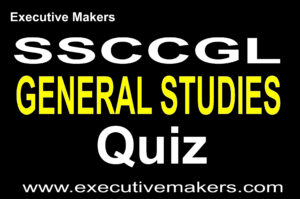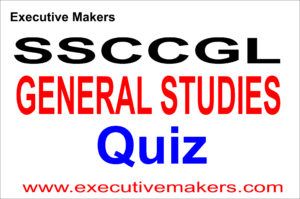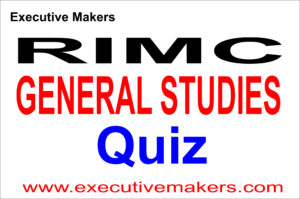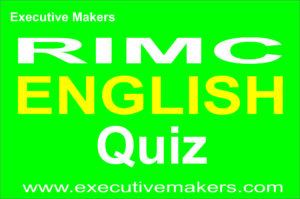# 40 Amazing Points of Science 10th Class

##### 40 Amazing Points of Science 10th Class

UNIFORM CIRCULAR MOTION(1) If an object moves on a circular path with a constant speed, its motion is said to be a uniform circular motion.

(2) In uniform circular motion, the speed of the body remains constant but direction changes continuously, due to which velocity changes continuously.

(3) There is an acceleration in the uniform circular motion which is always directed towards the centre of the circle. It is called centripetal acceleration.

(4) Centrifugal force (a pseudo force) is an apparent force in a certain situation that a body appears to be acted upon by a force while there is no force acting on the body.

##### 40 Amazing Points of Science 10th Class

MACHINES

(5) Machine is a device which acts as a force multiplier.

(6) Simple machines can be classified into two classes i.e. the lever and the inclined plane.

(7) Load (L) is the resistance overcome by the machine.

(8) Effort (E) is the force applied to overcome the resistance (or load).

##### 40 Amazing Points of Science 10th Class(9) The mechanical advantage of a machine is the ratio of the load to the effort.

(10) The velocity ratio of a machine is defined as the ratio of velocity of the effort to the velocity of the load.

Velocity Ratio (VR) = Velocity of effort / Velocity of load

(11) No machine works by itself. The work is to be done by the effort E on the machine which is called the work input.

Work Input = Effort (E) × Displacement of the effort (d)

(12) The work done by the machine in raising the load L is the work output.

##### 40 Amazing Points of Science 10th Class(13) For an ideal (Perfect) machine

Work output = Work input or Power output = Power input

(14) The efficiency of a machine is defined as the ratio of the useful work done by the machine to the total work put into the machine.

Efficiency = Output power / Input power

Efficiency = Work output / Work input

(15) The efficiency of an ideal machine is 1 or 100%. But for an actual machine, it is always less than 100%.

(16) Relation between efficiency, mechanical advantage and velocity ratio

Mechanical Advantage (MA) = Velocity ratio (VR) × Efficiency

##### 40 Amazing Points of Science 10th ClassLEVER

(17) A lever is a rigid, straight or bent bar which is capable of turning about a fixed turning point called fulcrum.

(18) According to principle of lever

(19) Depending upon the relative positions of the load, effort and fulcrum, levers are classified into three classes.

Class I lever = Fulcrum is between of Effort and Load

Class II lever = Load is between of Fulcrum and Effort

Class III lever = Effort is between of Fulcrum and Load

##### 40 Amazing Points of Science 10th Class(20) Remember FLE for fulcrum F, load L and effort E in between for Class I, II and III levers respectively.

(21) Remember

For class I lever = Effort arm may be greater, equal or less than Load एआरएम = MA and VR may be greater, equal or less than 1

For class II lever = Effort arm is greater than Load arm  = MA and VR are always greater than 1, hence act as a force multiplier

For class III lever = Effort arm is always less than Load arm = MA and VR are always less than 1, hence act as a speed multiplier

##### 40 Amazing Points of Science 10th Class

PULLEY

(22) Pulley is a wheel which is free to rotate on an axle.

For single fixed pulley, MA =1, VR = 1, Efficiency = MA/VR = 1 or 100%

For single movable pulley, MA = 2, VR = 2, Efficiency = MA/VR = 1 or 100%

##### 40 Amazing Points of Science 10th ClassGEARS

(23) Gears are a set of toothed wheels arranged together in a machine.

(24) In this arrangement, gear wheel closer to the source of power is called driver or driving gear while the gear wheel which receives motion from the driver is called as driven gear.

The number of teeth on a wheel depends on its circumference and hence, on its radius I.e.

N1/N2 = R1/R2

1 is for driving wheel and 2 is for driven wheel.

Where N is number of teeth on gear wheels and R is the radius of the wheel.

(25) Frequency (n) is inversely proportional to number of teeth (N) on the gear wheel i.e.

n is proportional to 1/N.

n1/n2 = N2/N1 = R2/R1

1 is for driving wheel and 2 is for driven wheel.

##### 40 Amazing Points of Science 10th Class

(26) Gear ratio is the ratio of number of teeth on the driving wheel (N1) to that on the driven wheel (N2) i.e. Gear ratio = N1/N2

If N1/N2 is greater than 1 then n2/n1 is also greater than 1. It means that the speed of rotation increases.

(Where N is the number of teeth and n is the frequency)

(27) A gear system is used to increase the speed if the driving gear has more number of teeth than the driven gear.

The gain in speed = Number of teeth in driving wheel / Number of teeth in driven wheel

(28) The gain in speed is the ratio of speed of rotation of driven wheel to the speed of rotation of driving wheel.

(29) Inclined Plane: This is a simple machine in which a rigid plank is kept inclined to the horizontal which helps to multiply the force.

##### 40 Amazing Points of Science 10th ClassWORK, ENERGY AND POWER

(30) Work is said to be done when a body moves in the direction of applied force.

(31) Work done is equal to the product of the force and distance.

W = F × s

(32) If the direction of applied force is not in the direction of motion of the body then

W = F cos α × s

Where F cos α is the component of force.

##### 40 Amazing Points of Science 10th Class

(33) Work is a scalar quantity.

(34) The SI unit of work is joule (J).

(35) 1 Joule of work is said to be done by a force of 1 newton when it displaces an object by 1 metre along the direction of force.

(36) Relation between the joule and the erg :

1 joule (J) = 107 erg

(37) An object which is able to do work is said to possess energy. The capacity of an object to do work is called its energy.

(38) Energy is a scalar quantity.

(39) The SI unit of energy is joule (J) and its CGS unit is erg.

(40) The larger units of energy are watt hour (W-h) and kilowatt hour (Kw-h).

1 Watt hour = 3600 J

1 Kilo watt hour = 3.6 × 106 joule

##### 40 Amazing Points of Science 10th Class

(41) Other units of energy are calories and e V.

1 cal = 4.18 J

1 e V = 1.6 × 10-19 J

(42) Power is the measure of how fast or slow work is done. It is defined as the rate of doing work.

Power (P) = Work done (W) / Time taken (t)

(43) Power is a scalar quantity. Its SI unit is joule/second and CGS unit is erg/second.

(44) In the honour of James Watt who developed steam engine, the SI unit of power is also called a watt (W).

1 Watt = 1 Joule/Second

##### 40 Amazing Points of Science 10th Class(45) Relation between SI and CGS units of power is

1 W = 1 J/S = 107 erg/s

1 KW = 1000 w = 103 Watt

1 MW (Mega Watt) = 106 Watt

1 GW (Giga Watt) = 109 Watt

(46) The practical unit of power used in engineering is horse power.

1 horse power (hp) = 746 watt (W)

#### https://executivemakers.com/product/v96-up-police-set-papers-pdf## Mail at : executivemakerspvtltd12@gmail.com